In the previous posts, I built the remote control with the 433MHz transceiver HC-12, which is unfortunately no longer in the range. This time, I would like to replace this with the nRF24L01 transceiver. This module can also be used as a transmitter and receiver under program control. But it is basically controlled differently, namely via the Serial Peripheral Interface, or SPI for short.

### Hardware

Number Component
1 Microcontroller board with ATmega328P, ATmega16U2, compatible with Arduino UNO R3

2 NRF24L01 with 2.4 GHz wireless module for Arduino, ESP8266, Raspberry Pi
possibly. Adapter for NRF24L01 (see note in text)
1 PS2 Joystick Shield Game Pad Keypad V2.0 for Arduino
or KY-023 Joystick Modul für Arduino UNO R3 u.a. MCUs
Breadboard, jumper cables, small items
possibly. Housing from the 3D printer

First, let's take a look at the nRF24L01 with its optional adapter.Even if I do not use the adapter for the nRF24L01 transceiver myself, I present it here, because firstly you can see the pin assignments very nicely.

Secondly, I have to give the following Note: The transceiver can only tolerate 3.3V voltage. For higher voltages, this adapter is recommended, where you can see the voltage regulator in the picture on the left.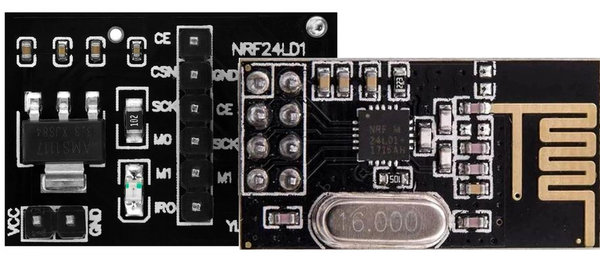From the designations of the pins you can quickly see that it is not connected to a serial interface (UART), but to the Serial Peripheral Interface (SPI).

Here is the typical pin assignment:

SCE Chip Enable (RX TX Mode Select) Digital D9
CSN Chip Select (Active Low) Digital D10
SCK Serial Clock Digital D13
MOSI Master Out Slave In Digital D11
MISO Master In Slave Out Digital D12
VCC 3,3V !!!
GND GND
IRQ not connected

You don't need to worry about this when you plug the nRF24L01 into the joystick shield. However, we have to remember SCE = 9 and CSN = 10, because we can and will define these pins ourselves, if necessary, while SCK, MOSI and MISO are permanently assigned.

For our sketch we need a new program library, which is added via the library manager. There we enter RF24 as the search term.If you scroll down a little you will find the TMRh20 library that I use.

As (almost) always, sample programs are also installed with the library. So scroll down under File / Examples to RF24. A sketch called GettingStarted is an obvious choice for first-time users.How nice that there are some explanations how to use at the beginning:

/**
* A simple example of sending data from 1 nRF24L01 transceiver to another.
*
* This example was written to be used on 2 devices acting as "nodes".
* Use the Serial Monitor to change each node's behavior.
*/

So: We need two MCUs each with the transceiver. The same sketch is uploaded to both MCUs; which micro controller is sender and which receiver is determined in the serial monitor.

Please remember that you have to start (instantiate) the Arduino IDE twice on a PC for two different virtual COM ports. It is not enough to open a new window.

And we need to make a small change in line 18. The pins for CE and CSN are defined there. The default is:

RF24 radio (7, 8); // using pin 7 for the CE pin, and pin 8 for the CSN pin

As indicated above, we change the pin numbers to CE / SCE = 9 and CSN = 10, that is

RF24 radio (9, 10); // using pin 9 for the CE pin, and pin 10 for the CSN pin

Compile, upload and try it out.

The nice thing about this program is that you get an error message if the transceiver does not react, e.g. because it was connected incorrectly or the above-mentioned change in the sketch was not made.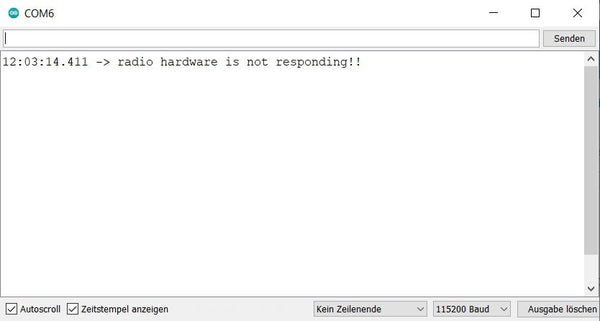With other programs where you don't get this feedback, troubleshooting often takes longer.

If everything is correct, you will be asked which device (node) it is. So enter 0 in the serial monitor of the first IDE for the first MCU and 1 in the other IDE .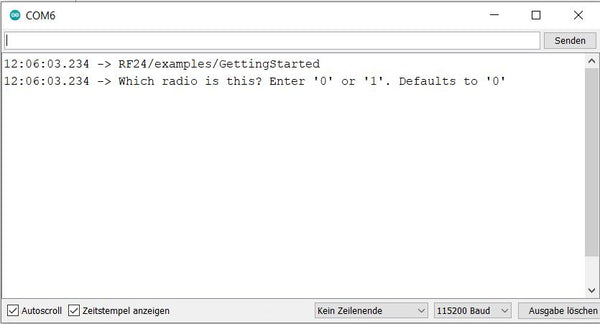Which node sends is decided by entering the letter T or t (for Transmit) in the top line of the Serial Monitor.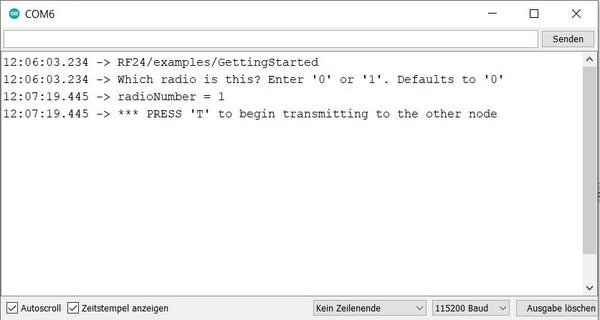I advise you to do these attempts in order to gain confidence in handling the transceivers. If something didn't work out in my attempts, I simply uploaded this sketch to see if I got the message "radio hardware not responding". Then the error was quickly found.

Now we are going to make the circuit and the program for our remote controls and the modified robot car, initially with two Nanos.

The Pin assignment is largely specified due to the SPI interface. When using the Nano, SCK is at pin D13, MISO at pin 12 and MOSI at pin 11. The other pins can be selected. I choose CE on pin D2 and CSN on pin D3 so as not to occupy any PWM pins that are used for the motors in the second sketch. For the joystick I use the analog inputs A6 and A7 as well as D4 for the button.

Picture and sketch for remote control: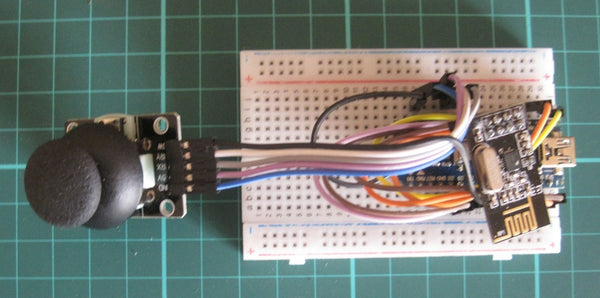/*
Joystick als Motor Controller, Stillstand = 505
je 5 Stufen vor/zurück, je 5 Stufen rechts/links
*
* Library: TMRh20/RF24, https://github.com/tmrh20/RF24/
*/
#include <SPI.h>
#include <nRF24L01.h>
#include <RF24.h>
RF24 radio(2, 3); // CE, CSN
const byte address = "CodeR";

int x = 5; // x-Achse = links/rechts
int y = 5; // y-Achse = vor/zurück
int code = 505;
int joybutton = 4; // Joystick button
float faktor = 1.0; // für Anpassung bei unterschiedlicher Spannung oder ADC

void setup() {
pinMode(joybutton,INPUT_PULLUP);
Serial.begin(115200);
radio.begin();
radio.openWritingPipe(address);
radio.setPALevel(RF24_PA_MIN);
radio.stopListening();
}

void sendcode() {
radio.write(&code, sizeof(code));
delay(100); // little delay for next button press
}

void loop() {
float A6 = faktor * analogRead (6);
float A7 = faktor * analogRead (7);
bool button = digitalRead(joybutton);

Serial.print("x-Achse: ");
Serial.print(A6);
Serial.print("y-Achse: ");
Serial.print(A7);
Serial.print(" Button pressed ");
Serial.print(button);

x = int(A6/100);
y = int(A7/100);
code = 100*y + x;
Serial.print(" Code = ");
Serial.println(code);
sendcode();
}

And here picture and sketch for the robot car with two motors: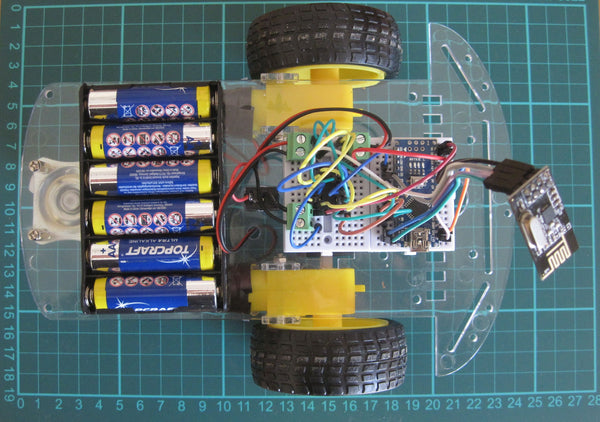#include
#include
#include
RF24 radio (2, 3); // CE, CSN
const byte address  = "CodeR";

// define variables for speed control
int x = 0;
int y = 0;
int left = 0;
int right = 0;
int code = 505;
int speedL = 0;
float factor = 0.66; // correction of speedLevel = maxValue / 255
// define motor pins
int m11 = 5; // left motor (s) pwr1
int m12 = 6; // left motor (s) pwr2
int m1e = 7; // left motor (s) enable

int m21 = 9; // right motor (s) pwr1
int m22 = 10; // right motor (s) pwr2
int m2e = 8; // right motor (s) enable

void setup () {
Serial.begin (9600); // set up Serial Monitor at 9600 bps
Serial.println ("Motor test!");

// initialize radio nRF24L01
radio.begin ();
radio.openReadingPipe (0, address);
radio.setPALevel (RF24_PA_MIN);
radio.startListening ();

// initialize digital pins as output for motors.
pinMode (m11, OUTPUT);
pinMode (m12, OUTPUT);
pinMode (m1e, OUTPUT);
digitalWrite (m1e, LOW);
pinMode (m21, OUTPUT);
pinMode(m22, OUTPUT);
pinMode(m2e, OUTPUT);
digitalWrite(m2e, LOW);

} // end of setup

void loop() {

if (radio.available()) {
code = 0;
radio.read(&code, sizeof(code));
Serial.println(code);
motor();
}
delay(20); //little delay for better serial communication

} // end of loop

void motor(){
int speedLevel={-255,-210,-165,-120,-75,0,75,120,165,210,255};
y = int(code /100);
x = code - 100*y;
speedL = speedLevel[y];
Serial.print("code = ");
Serial.print(code);
Serial.print(" y = ");
Serial.print(y);
Serial.print(" x = ");
Serial.print(x);
Serial.print(" speedL = ");
Serial.println(speedL);

//correction of speedLevel for cornering
if (x==0){
right = speedL+60;
left = speedL-60;
}
else if (x==1){
right = speedL+48;
left = speedL-48;
}
else if (x==2){
right = speedL+36;
left = speedL-36;
}
else if (x==3) {
right = speedL+24;
left = speedL-24;
}
else if (x==4) {
right = speedL+12;
left = speedL-12;
}
else if (x==6) {
right = speedL -12;
left = speedL+12;
}
else if (x==7) {
right = speedL-24;
left = speedL+24;
}
else if (x==8) {
right = speedL-36;
left = speedL+36;
}
else if (x==9) {
right = speedL-48;
left = speedL+48;
}
else if (x==10) {
right = speedL-60;
left = speedL+60;
}
else {
right = speedL;
left = speedL;
}

//speedLevel for "left" and "right"
Serial.print("left = ");
Serial.print(left);
Serial.print(" right = ");
Serial.println(right);

// stop
if (left < 63 & left > -63) {
digitalWrite(m1e, LOW);
}
if (right < 63 & right > -63) {
digitalWrite(m2e, LOW);
}
// forward
if (left>=63) {
if (left>255) left=255;
int corrl=int(left * factor);
Serial.print("corrl = ");
Serial.println(corrl);
analogWrite(m11,corrl);
analogWrite(m12, 0);
digitalWrite(m1e, HIGH);
}
if (right>=63) {
if (right>255) right=255;
int corrr=int(abs(right) * factor);
Serial.print("corrr = ");
Serial.println(corrr);
analogWrite(m21, corrr);
analogWrite(m22, 0);
digitalWrite(m2e, HIGH);
}
// backward
if (left<= -63) {
if (left<-255) left=-255;
int corrl=int(abs(left) * factor);
Serial.print("corrl = ");
Serial.println(corrl);
analogWrite(m11,corrl);
analogWrite(m11, 0);
analogWrite(m12, int(abs(left) * factor));
digitalWrite(m1e, HIGH);
}
if (right<= -63) {
if (right<-255) right=-255;
int corrr=int(abs(right) * factor);
Serial.print("corrr = ");
Serial.println(corrr);
analogWrite(m21, 0);
analogWrite(m22, corrr);
digitalWrite(m2e, HIGH);
}
Serial.print("Motorsteuerung okay");
} // end of motor

As you can see in the picture with the flying cables, the nRF23L01 - and also the adapter - is not suitable for being plugged onto the breadboard, so here is another picture of the joystick shield with a slot for the nRF24L01.As a reminder: Here the pin assignment was CE = Pin 9, CSN = Pin10, x-axis = A0, y-axis = A1 and JoyStick button = D8. With these changes, the Sketch Motor Controller works.

Overall, the nRF24L01 transceiver is an inexpensive and good solution for the radio remote control of our little robot cars.

In the next blog posts we will expand the control with sensors to get a speed controller and finally also to be able to drive autonomously.

For arduino

2 commentsWalter

Mit Arduino UNO konnte ich den nRF24L01 nicht zum laufen bringen, zig Internet Beiträge zum trotz.

Da entgegen klappte es mit Arduino NANO und sogar mit ESP32 Dev Kit auf Anhieb.Rudi Brand

Hi, klingt gut! Wäre das eine Möglichkeit, einen Dashbutton-Ersatz zu bauen? Senden direkt auf Tastendruck in einem Batteriebetriebenen Gehäuse und der Empfänger am Netzteil sendet dann per MQTT ins private Netz? Aktuell habe ich einige Buttons mit ESP8266 gebaut, die im Deepsleep sind und nach dem Drücken 3-5s brauchen, bis sie im WLAN sind und mit die Batteriespannung per MQTT liefern..

Leave a comment

All comments are moderated before being published

Recommended products

Main menu